The K5 Learning Blog urges parents to be pro-active in helping their children reach their full academic potential.

K5 Learning
provides free worksheets, workbooks and an online reading and math program for kindergarten to grade 5 students.# Proportions Word Problems with Answers

In grade 6 students learn about ratios and proportions. We’ve created a series of proportions word problems worksheets and ratio word problems worksheets for students to practice.

Let’s take a more detailed look at how to solve proportions word problems.

## Proportions

First, what are proportions? Proportion means that two ratios (or fractions) are equal.

For example: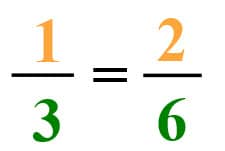1 out of 3 is equal to 2 out of 6. The ratios are the same, so they are in proportion.

In a real-world example, you could apply it this way.

A ball of yarn’s length and weight are in proportion.100 meter of yarn weighs 3 ounces.200 meter of yarn weighs 6 ounces.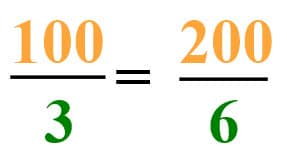## Proportions word problem

We’re making lemonade. If you mix 3 liters of water with 4 lemons to make lemonade, how many liters of water do you need for 8 lemons?

Let’s set up the ratios. We know they involve lemons and liters of water, like this: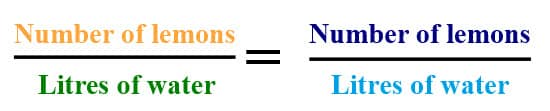We know the following from word problem. We’ll use an x for the unknown number: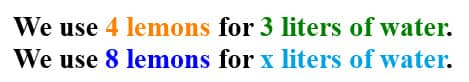Now we can place the numbers we know in the correct places in the equation.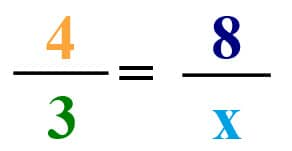We multiply across the known corners: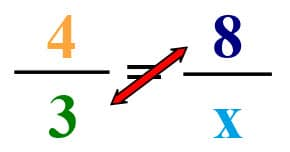3 x 8 = 24

As this is a proportion, the sum of 4 x X also has to be 24.

Now, you can either divide 24 by 4, or you can write out the equation:

4 x X = 24. From learning our math facts, we know that 4 x 6 = 24.

Therefore, we need 6 liters of water for making lemonade with 8 lemons.

We’ve created two sets of free worksheets to practice proportions word problems:

Proportions word problems

Proportions word problems – using decimals